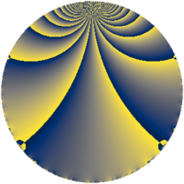# Properties

 Label 784.4.bbLevel $784$ Weight $4$ Character orbit 784.bb Rep. character $\chi_{784}(111,\cdot)$ Character field $\Q(\zeta_{14})$ Dimension $504$ Sturm bound $448$

# Related objects

## Defining parameters

 Level: $$N$$ $$=$$ $$784 = 2^{4} \cdot 7^{2}$$ Weight: $$k$$ $$=$$ $$4$$ Character orbit: $$[\chi]$$ $$=$$ 784.bb (of order $$14$$ and degree $$6$$) Character conductor: $$\operatorname{cond}(\chi)$$ $$=$$ $$196$$ Character field: $$\Q(\zeta_{14})$$ Sturm bound: $$448$$

## Dimensions

The following table gives the dimensions of various subspaces of $$M_{4}(784, [\chi])$$.

Total New Old
Modular forms 2052 504 1548
Cusp forms 1980 504 1476
Eisenstein series 72 0 72

## Trace form

 $$504q - 756q^{9} + O(q^{10})$$ $$504q - 756q^{9} - 144q^{21} + 1596q^{25} + 420q^{29} - 1260q^{37} + 948q^{49} + 1176q^{53} - 168q^{57} - 3780q^{61} - 840q^{65} - 10920q^{69} - 2136q^{77} - 2184q^{81} + 1176q^{85} - 1512q^{93} + O(q^{100})$$

## Decomposition of $$S_{4}^{\mathrm{new}}(784, [\chi])$$ into newform subspaces

The newforms in this space have not yet been added to the LMFDB.

## Decomposition of $$S_{4}^{\mathrm{old}}(784, [\chi])$$ into lower level spaces

$$S_{4}^{\mathrm{old}}(784, [\chi]) \cong$$ $$S_{4}^{\mathrm{new}}(196, [\chi])$$$$^{\oplus 3}$$